﻿ Set Difference | Difference of Two Sets Calculator | Calculators4U

# Set Difference - Difference of Two Sets Calculator

In the set operations, let's calculate set difference. The difference of two set A and set B, denoted by A - B, is the set that contains exactly all elements in A but not in B.

### Set Difference

The set operation difference between sets implies subtracting the elements from a set which is similar to the concept of the difference between numbers. The difference between sets A and B denoted as A − B lists all the elements that are in set A but not in set B. To understand this set operation of set difference better, let us consider an example: If A = {1, 2, 3, 4} and B = {3, 4, 5, 6}, then the difference between sets A and B is given by A - B = {1, 2}.

### Set Operations Table

Set OperationVenn DiagramInterpretation
Union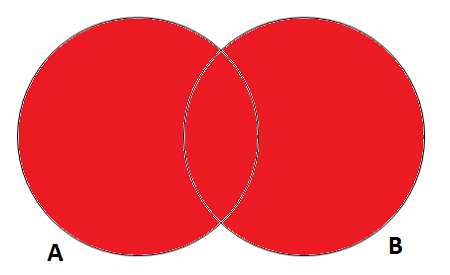A U B, is the set of all values that are a member of A, or B, or both.
Intersection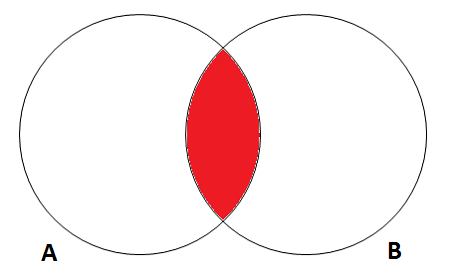A ∩ B, is the set of all values that are a member of both A and B.
Difference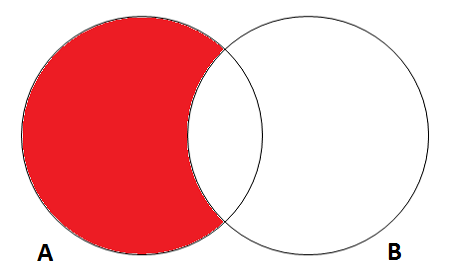A \ B, is the set of all values of A that are not members of B.
Symmetric Difference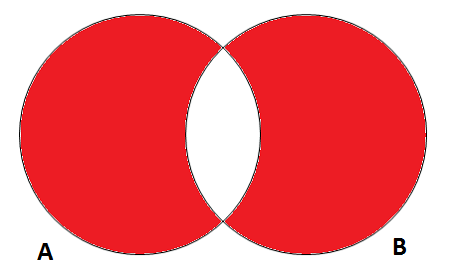A ∆ B, is the set of all values which are in one of the sets but not both.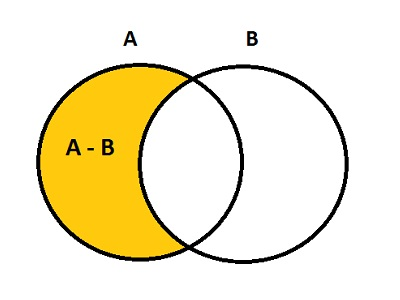### Spreading Knowledge Across the World

USA - United States of America  Canada  United Kingdom  Australia  New Zealand  South America  Brazil  Portugal  Netherland  South Africa  Ethiopia  Zambia  Singapore  Malaysia  India  China  UAE - Saudi Arabia  Qatar  Oman  Kuwait  Bahrain  Dubai  Israil  England  Scotland  Norway  Ireland  Denmark  France  Spain  Poland  and  many more....